# Basic Properties of Definite Integrals

Go back to  'Definite Integration'

## Properties of definite integrals

As explained in the chapter titled “Integration Basics”, the fundamental theorem of calculus tells us that to evaluate the area under a curve $$y = f\left( x \right)$$ from $$x = a\,\,{\rm{to}}\,\,x = b$$ , we first evaluate the anti-derivative $$g\left( x \right)\,\,{\rm{of}}\,f\left( x \right)$$

$g\left( x \right)= \int {f\left( x \right)dx}$

and then evaluate $$g\left( b \right) - g\left( a \right).$$ That is, area under the curve f(x) from $$x = a$$ to $$x = b$$ is

$\int\limits_a^b {f\left( x \right)dx = g\left( b \right) - g\left( a \right)}$

Readers who have even the slightest doubt regarding the discussion above are advised to refer to the chapter on “Integration Basics” before reading on.

Definite integration is not all about just evaluating the anti-derivative and substituting the upper and lower limits. Working through this chapter, you will realise that a lot of techniques exist which help us in evaluating the definite integral without resorting to the (many times tedious) process of first determining the anti-derivative. We will develop all these techniques one by one from scratch, starting with some extremely basic properties in Section – 1

# Section - 1  BASIC PROPERTIES

(1) Suppose that f(x) < 0 on some interval [a, b]. Then, the area under the curve y = f(x) from $$x = a$$ to $$x = b$$ will be negative in sign, i.e

$\int\limits_a^b {f\left( x \right)dx < 0}$

This is obvious once you consider how the definite integral was arrived at in the first place; as a limit of the sum of the n rectangles $$\left( {n \to \infty } \right)$$ . Thus, if f(x) < 0 in some interval then the area of the rectangles in that interval will also be negative.

This property means that for example, if $$f(x)$$ has the following form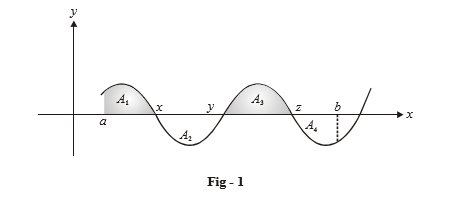then $$\int\limits_a^b {f\left( x \right)dx\,\,{\rm{will}}\,\,{\rm{equal}}\,{A_1} - {A_2} + {A_3} - {A_4}\,\,{\rm{and}}\,\,{\rm{not}}\,} {A_1} + {A_2} + {A_3} + {A_4}.$$

If we need to evaluate $${A_1} + {A_2} + {A_3} + {A_4}$$ (the magnitude of the bounded area), we will have to calculate

$\int\limits_a^x {f\left( x \right)dx + \left| {\int\limits_x^y {f\left( x \right)dx} } \right|} + \int\limits_y^z {f\left( x \right)dx + \left| {\int\limits_z^b {f\left( x \right)dx} } \right|}$

From this, it should also be obvious that $$\left| {\int\limits_a^b {f\left( x \right)dx} } \right| \le \,\,\int\limits_a^b {\left| {f\left( x \right)} \right|dx}$$

(2) The area under the curve y = f(x) from x = a to x = b is equal in magnitude but opposite in sign to the area under the same curve from x = b to x = a, i.e

$\int\limits_a^b {f\left( x \right)dx} = - \int\limits_b^a {f\left( x \right)dx}$

This property is obvious if you consider the Newton-Leibnitz formula. If $$g\left( x \right)$$ is the anti-derivative of x(f), then $$\int\limits_a^b {f\left( x \right)} \,dx$$ is $$g\left( b \right) - g\left( a \right)\,\,{\rm{while}}\,\,\int\limits_b^a {f\left( x \right)dx} \,\,{\rm{is}}\,\,g\left( a \right) - g\left( b \right)$$ .

(3) The area under the curve y = f(x) from x = a to x = b can be written as the sum of the area under the curve from x = a to x = c and from x = c to x = b, that is

$\int\limits_a^b {f\left( x \right)dx = \int\limits_a^c {f\left( x \right)dx + \,} \int\limits_c^b {f\left( x \right)dx} }$

Let us consider an example of this. Let $$c \in (a,\,\,b)$$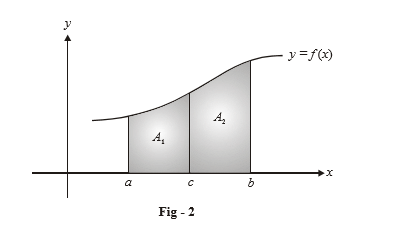It is clear that the area under the curve from x = a to x = b, A is  $${{A}_{1}}+{{A}_{2}}$$

Note that c need not lie between a and b for this relation to hold true. Suppose that c > b.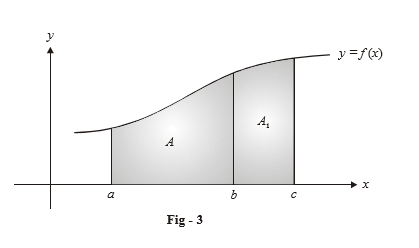Observe that $$A = \int\limits_a^b {f\left( x \right)dx = \left( {A + {A_1}} \right) - {A_1}}$$

$\begin{array}{l}= \int\limits_a^c {f\left( x \right)dx - \int\limits_b^c {f\left( x \right)dx} } \\= \int\limits_a^c {f\left( x \right)dx + \int\limits_c^b {f\left( x \right)dx} }\end{array}$

Analytically, this relation can be proved easily using the Newton Leibnitz’s formula.

(4) Let $$f\left( x \right) > g\left( x \right)$$ on the interval [a, b]. Then,

$\int\limits_a^b {f\left( x \right) > \int\limits_a^b {g\left( x \right)} \,dx.}$

This is because the curve of f(x) lies above the curve of g(x), or equivalently, the curve of $$f\left( x \right) - g\left( x \right)$$ lies above the x-axis for [a, b]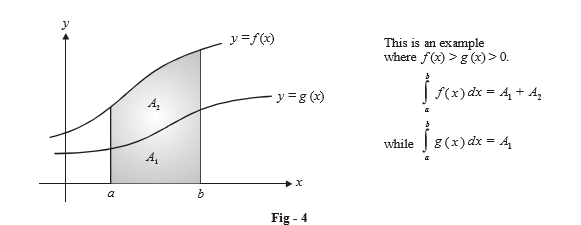Similarly, if $$f\left( x \right) < g\left( x \right)\,$$ on the interval [a, b], then

$\int\limits_a^b {f\left( x \right)dx < } \,\int\limits_a^b {g\left( x \right)dx}$

(5) For the interval [a, b], suppose m < f(x) < M. That is, m is a lower-bound for f(x) while M is an upper bound.

Then,

$m\left( {b - a} \right)\,\, < \int\limits_a^b {f\left( x \right)dx\,\, < \,\,\,M\left( {b - a} \right)}$

This is obvious once we consider the figure below: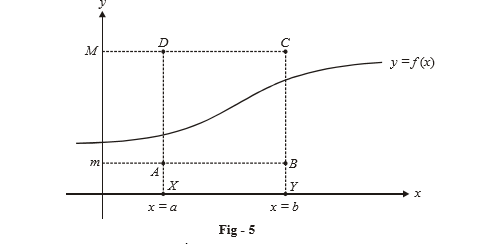Observe that $${\rm{area}}\left( {{\rm{rect}}\,AXYB} \right) < \int\limits_a^b {f\left( x \right)dx < {\rm{area}}\left( {{\rm{rect}}\,DXYC} \right)}$$

(6) Let us consider the integral of $${f_1}\left( x \right) + {f_2}\left( x \right)\,\,{\rm{from}}\,\,x = a\,\,{\rm{to}}\,\,x = b$$ . To evaluate the area under $${f_1}\left( x \right) + {f_2}\left( x \right),$$ we can separately evaluate the area under  $${{f}_{1}}(x)$$ and the area under $${{f}_{2}}(x)$$ and add the two areas (algebraically). Thus:

$\int\limits_a^b {\left( {{f_1}\left( x \right) + {f_2}\left( x \right)} \right)\,dx\,\, = } \,\,\int\limits_a^b {{f_1}\left( x \right)\,dx\,\, + } \,\,\int\limits_a^b {{f_2}\left( x \right)\,dx}$

Now consider the integral of kf(x) from x = a to x = b. To evaluate the area under kf(x), we can first evaluate the area under f(x) and then multiply it by k, that is:

$\int\limits_a^b {kf\left( x \right)dx\,\, = \,\,} k\int\limits_a^b {f\left( x \right)dx}$

(7) Consider an odd function f(x), $$i.e,f\left( x \right) = - f\left( { - x} \right).$$ This means that the graph of f(x) is symmetric about the origin.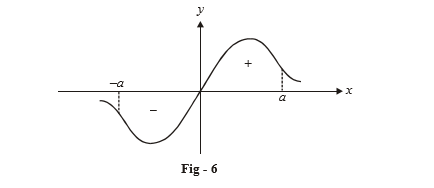From the figure, it should be obvious that $$\int\limits_{ - a}^a {f\left( x \right)dx = 0,}$$ because the area on the left side and that on the right algebraically add to 0.

Similarly, if f(x) was even, $$i.e,\,\,f\left( x \right) = f\left( { - x} \right)$$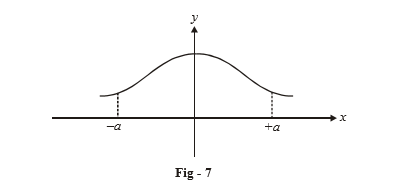$$\int\limits_{ - a}^a {f\left( x \right)dx = \,\,2} \int\limits_0^a {f\left( x \right)\,\,dx}$$ because the graph is symmetrical about the y-axis.

If you recall the discussion in the unit on functions, a function can also be even or odd about any arbitrary point x = a. Let us suppose that f(x) is odd about x = a, i.e

$f\left( x \right) = - f\left( {2a - x} \right)$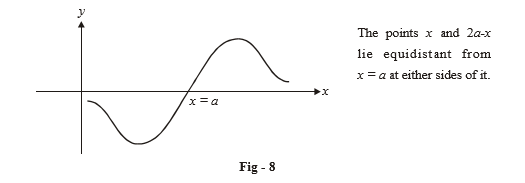Suppose for example, that we need to calculate $$\int\limits_0^{2a} {f\left( x \right)dx.}$$ It is obvious that this will be 0, since we are considering equal variation on either side of x = a, i.e. the area from x = 0 to x = a and the area from x = a to x = 2a will add algebraically to 0.

Similarly, if f(x) is even about x = a, i.e.

$f\left( x \right) = f\left( {2a - x} \right)$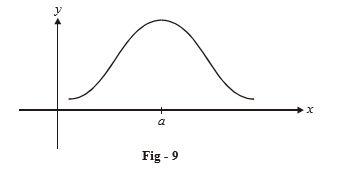then we have, for example

$\int\limits_0^{2a} {f\left( x \right)dx = 2\int\limits_0^a {f\left( x \right)dx} }$

From this discussion, you will get a general idea as to how to approach such issues regarding even/odd functions.

(8) Let us consider a function f(x) on [a, b]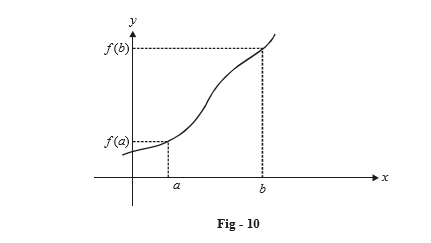We want to somehow define the “average” value that f(x) takes on the interval [a, b]. What would be an appropriate way to define such an average?

Let $${f_{av}}$$ be the average value that we are seeking. Let it be such that it is obtained at some $$x = c\,\, \in [a,\,\,b]$$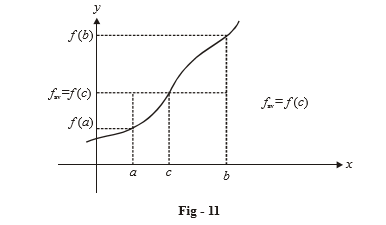We can measure $${f_{av}}$$ by saying that the area under f(x) from x = a to x = b should equal the area under the average value from x = a to x = b. This seems to be the only logical way to define the average (and this is how it is actually defined!). Thus

$\begin{array}{l} \,\,\,\,\,\,\,\,{f_{av}}\left( {b - a} \right) = \int\limits_a^b {f\left( x \right)dx} \\\Rightarrow {f_{av}} = \frac{1}{{b - a}}\int\limits_a^b {f\left( x \right)dx}\end{array}$

This value is attained for at least one $$c \in \left( {a,\,\,b} \right)$$ (under the constraint that f is continuous, of course).